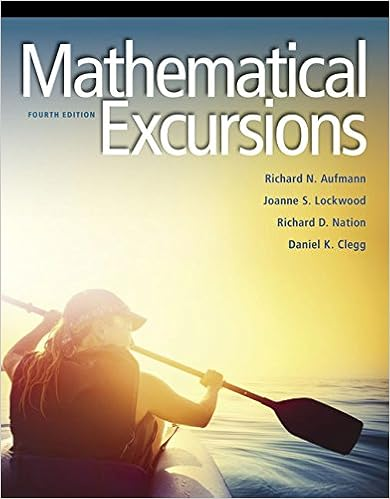# Test-3-Spring-2016 - MA 114-002 Test 3 Answer the questions...

• Test Prep
• 2
• 100% (1) 1 out of 1 people found this document helpful

This preview shows page 1 - 2 out of 2 pages.

##### We have textbook solutions for you!
The document you are viewing contains questions related to this textbook.The document you are viewing contains questions related to this textbook.
Chapter 1 / Exercise 15
Mathematical Excursions
AufmannExpert Verified
MA 114-002 Test 3 March 16, 2016 Answer the questions in the blue book provided. You must show all of your work to receive full credit. Only non-graphing calculators are allowed for this exam. You may leave your answer as C p n, k q for appropriate n and k . 1. 2 points In the following questions, indicate whether the statement is true or false. If it is false, provide a counter-example. (a) S is a subset of S Y T (b) S X T is a subset of T 1 2. In the following problems, draw a three-circle Venn diagram and label the circles R , S , and T . Shade the portion of the diagram corresponding to the given set. (a) 2 points R Y S 1 Y T 1 (b) 3 points R 1 X p S Y T q 3. Suppose that S and T are subsets of the universal set U . Draw a two-circle Venn diagram, and use the use given information to fill in the number of elements in each of the non-overlapping regions of the diagram. (a) 2 points n p U q “ 15, n p S q “ 6, n p T q “ 8, n p S X T q “ 2 (b) 3 points n p U q “ 75, n p S q “ 15, n p T q “ 25, and n p S 1 X T 1 q “ 40. 4. 2 points
##### We have textbook solutions for you!
The document you are viewing contains questions related to this textbook.The document you are viewing contains questions related to this textbook.
Chapter 1 / Exercise 15
Mathematical Excursions
AufmannExpert Verified
•••